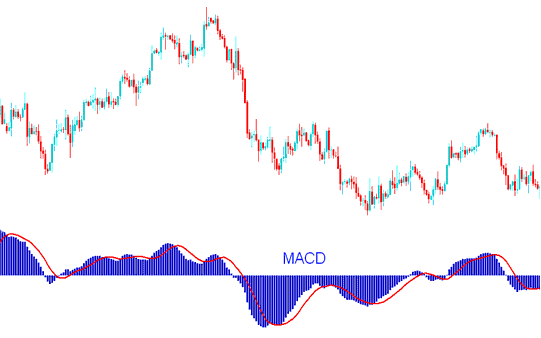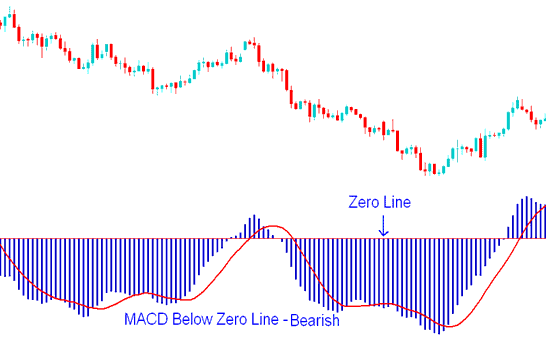# MACD Center Line Crossover - Bullish and Bearish Forex Trading Signals

The MACD forex indicator is one of the most widely and commonly used forex indicators available. MACD indicator is a momentum oscillator with some trend following characteristics.

MACD is one of the most popular indicators used in forex technical analysis. MACD indicator is used to generate forex trading signals using crossovers.

MACD plots the divergence and convergence of moving averages. MACD indicator is constructed using moving average analysis. Moving Average Convergence/Divergence is a trend-following forex technical indicator. MACD indicator shows the correlation between two moving averages.

One moving average is of a shorter period and the other for a longer period of price bars.MACD Indicator - MACD Forex Trading Indicator Technical Analysis

MACD forex indicator has a zero center line; values above zero line are bullish while those below zero are bearish.

In an upward forex trend the shorter MACD line rises faster that the longer MACD line this creates a gap. In addition as long as the MACD indicator is above the center mark the trend is still bullish as shown below.

Do not sell as long as the MACD Forex Indicator is above the Center Mark - this is bullish territory and it does not matter how it is moving as long as it is above the zero center mark, as shown on the example below.MACD Indicator Above Zero Mark - Bullish Forex Trading Signal

In a downward forex trend the shorter MACD line falls faster than the longer MACD line this creates a gap. In addition as long as the indicator is below the center mark the trend is still bearish as shown below.

Do not buy as long as the MACD Forex Indicator is below the Center Mark - this is bearish territory and it does not matter how it is moving as long as it is below the zero center mark, as shown on the example below.MACD Indicator Below Zero Center Line Mark - Bearish Forex Trading Signal

When the forex trend is about to reverse the MACD lines start to move closer to each other, thus closing the gap.